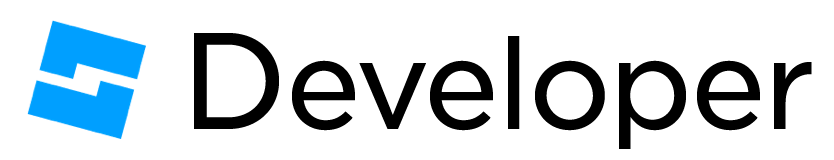# How can I detect if a part is surrounded by other parts?

1 Like

I believe that if the parts are touching, you can do `MainPart:GetTouchingParts()` which returns an array of the touching parts. If that doesn’t work, you could use Region3 and FindPartsInRegion3.

2 Likes

I’m not sure I completely understand your question are you trying to check if a certain amount of parts surround a part In general or parts that are directly touching a part or within a confined area?

if your looking for parts that directly surround a part GetTouchingParts as mentioned above should work

1 Like

I want to know if all surfaces of the part is surrounded by other parts. And the other parts will be touching the part.

1 Like

if you had a grid principle in mind, that would be easy, simply raycast in all 6 directions and see if there is a part intersecting each ray

2 Likes

Last question(sorry) do you need to detect if all parts that surround a part to fully cover the surface it’s “on”, so that basically the main part isn’t visible?

1 Like

transparency isn’t part of collision but CanCollide is, see Simple trick to make GetTouchingParts work with non-CanCollide parts .

And if you mean other game-specific logic, you can work that out in multiple ways, for example having a BoolValue in each part and seeing if its value is true or false to determine on or off.

1 Like

It does not need to fully cover the surface, but It does need to touch the surface.

Will your parts always be cubes, will they always be aligned within a grid, and will they always be the same size?

It should be noted that :GetTouchingParts() does not return adjacent parts, meaning that if they only share a surface it will not return the adjacent part using that function. Now, you can either do raycasting for this as mentioned earlier, or use a custom function like the one outlined in the article below.

The article below outlines a custom function for finding adjacent parts, this might apply better to what you are doing.

Cheers, hope my answer helped a bit!

1 Like

yes… I’m making a voxel game…

If this is a voxel game, then this can be solved using a 3D array Assuming your map doesn’t get too large you can use an algorithm to check all around a specific point of a 3D array. Each index will be a component of the location of the part and we can store a string for a block id if it exists, or nil if there is no object there.

Like so:

``````--Here is our 3D array
local Array3D

--Here is where we make a blank map if you would
local xLength = 10
local yLength = 10
local zLength = 10

Array3D = table.create(xLength)
for x=1, xLength do
local dimY = table.create(yLength)
Array3D[x] = dimY
for y=1, yLength do
dimY[y] = table.create(zLength) --Here is what our default values are, in this case nil.
end
end

--Here is an example of getting an object at x=1, y=2, z=3
local object = Array3D

--Here is an example of setting an object at x=3, y=4, z=8
Array3D = "dirt"

--Here we create a function test around a given point to see if it is surrounded by blocks and track which faces are occupied
--It returns in the order of   up, down, left, right, front, back
local function getFacesOccupied(x, y, z)
local up, down, left, right, front, back = false, false, false, false, false, false

--Ensure Bounds
if x <= xLength and x >= 1 and y <= yLength and y >= 1 and z <= zLength and z >= 1 then

if x-1 > 0 and x+1 <= xLength then
--Test left face
if Array3D[x+1][y][z] ~= nil then
left = true
--Test right face
elseif Array3D[x-1][y][z] ~= nil then
right = true
end
end

if y-1 > 0 and y+1 <= yLength then
--Test top face
if Array3D[x][y+1][z] ~= nil then
up = true
--Test bottom face
elseif Array3D[x][y-1][z] ~= nil then
down = true
end
end

if z-1 > 0 and z+1 <= zLength then
--Test front face
if Array3D[x][y][z+1] ~= nil then
front = true
--Test back face
elseif Array3D[x][y][z-1] ~= nil then
back = true
end
end

end

return up, down, left, right, front, back
end

--Here we get faces occupied at x=2, y=4, z=5
local up, down, left, right, front, back = getFacesOccupied(2, 4, 5)
``````

Note that in this example all parts were at a 1, 1, 1 size, if you want to make parts at a 4, 4, 4 size, you can simply just use the each component and multiply it by 4 to get the position. Example:

``````local partSize = 4
local x, y, z = 1, 1, 1

SomePart.Size = Vector3.new(partSize, partSize, partSize)
SomePart.Position = Vector3.new(x*partSize , y*partSize , z*partSize)
``````
2 Likes